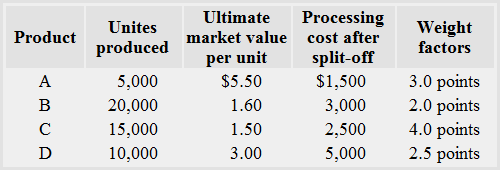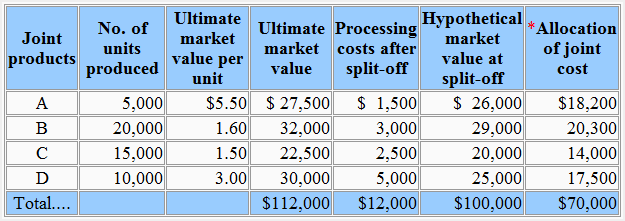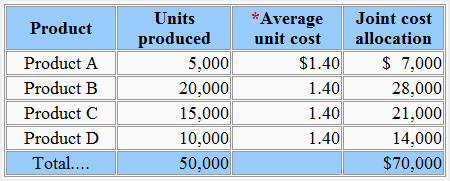Problem-4: Three methods of allocating joint costs

The Sun Inc. produces four joint products – product A, product B, product C and product D. The joint production cost at split-off point is \$70,000. The data for the month of April is given below:Required: Allocate joint production cost using:

1. The market value method
2. The average unit cost method
3. The weighted average method

Solution

1. The market value method:*The joint cost is 50% of the hypothetical market value.

2. Average unit cost method:*Joint cost/Total number of units produced
= \$70,000/50,000
= \$1.40 per unit

3. Weighted average method:*Joint cost/Total number of weighted units
= \$70,000/140,000
= \$.50 per weighted unit

Show your love for us by sharing our contents.